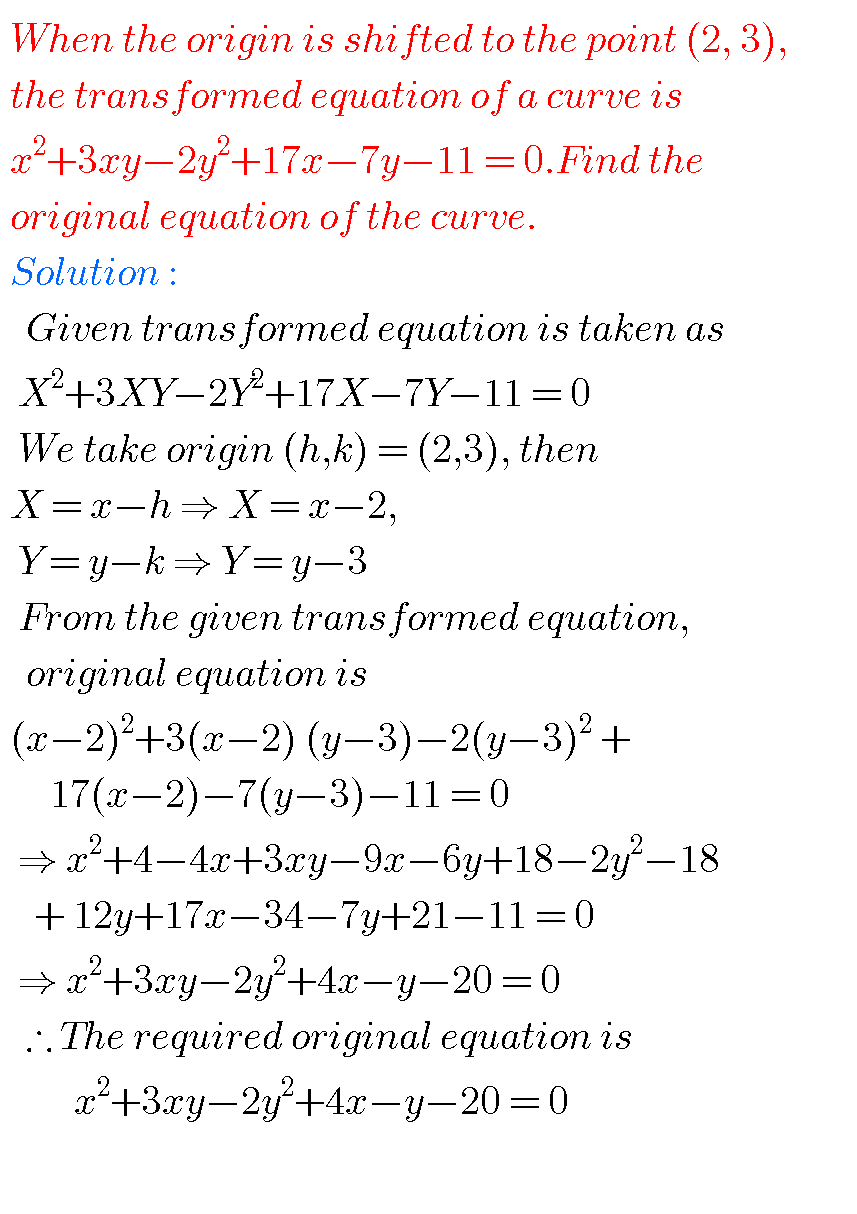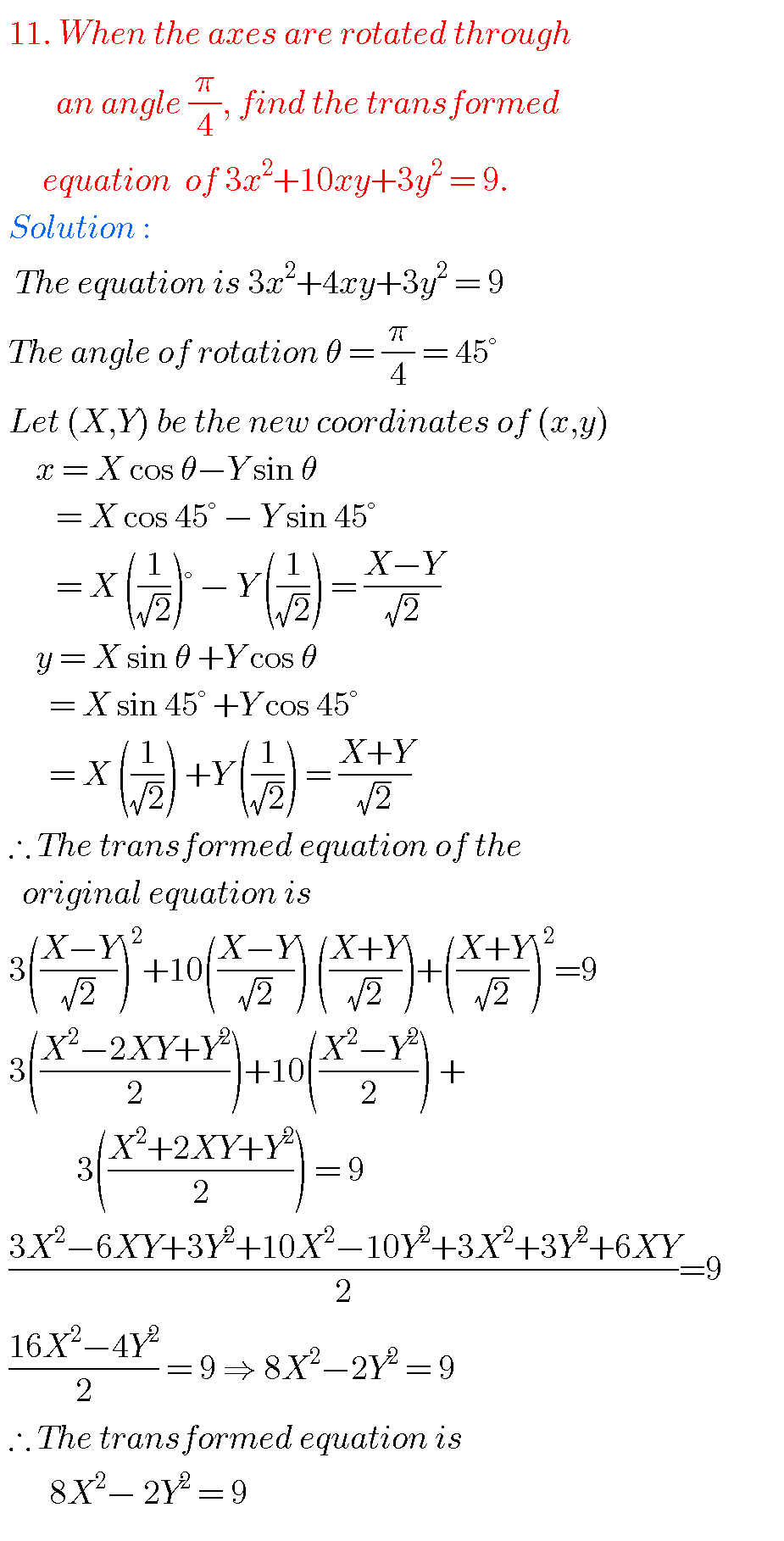# TRANSFORMATION OF AXES Inter, INTERMEDIATE FIRST YEAR 1B SOLUTIONS

Mathematics Intermediate1A Transformation of axes solutions for examination purpose are given below.

These are very simple to understand.

Study the textbook lesson Transformation of axes very well.

Observe the example problems and solutions given in the textbook.

Transformation of axes textbook solutions

Exercise 2(a)

You can also see

Inter Maths 1A textbook solutions

Inter Maths 1B textbook solutions

Inter Maths IIA textbook solutions

Inter Maths IIB textbook solutions

M

## TRANSFORMATION OF AXES, INTERMEDIATE 1ST YEAR 1B CHAPTER 2 PROBLEMS WITH SOLUTIONSProblem 24

Problem 56Problem 8Problem 9

###Problem 10### TRANSFORMATION OF AXES, INTERMEDIATE FIRST YEAR 1B CHAPTER 2 PROBLEMS WITH SOLUTIONSNote ; Observe the solutions and try them in your own methods.

Some moreInter maths trigonometry solutions

Maths real numbers solutions class 10

You can also see the solution of junior inter maths 1B coordinate geometry

1Locus

Straight lines sa

Straight lines la

12. Rate measure

### 7 thoughts on “Transformation of Axes,Intermediate first year 1B chapter 2 problems with solutions”

1.It’s really helpful but i insist you to note the formulae wherever you use them.

2.very helpful and quick revision of imp question , thanku

3.It’s really fantastic and very helpful but give the problems in order wise according to text book so it will become easy to find the problems

4.It’s really very helpful during these online classes

5.lokesh vangapandu

online class is so nice so helpful and fantastic

6.It’s very useful to us during online classes

7.good😊